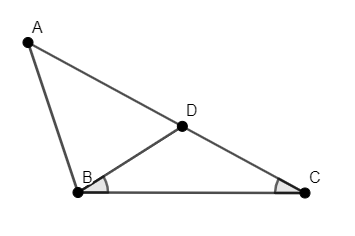QUESTION

# Check if the given statement is correct or not: In any triangle, the side opposite to the larger (greater) angle is longer.(a) True(b) False

Hint: Consider any triangle $\Delta ABC$ such that $\angle B>\angle C$. Use the fact that the angles opposite to equal sides are equal and geometric construction to check if the given statement is correct or not. Another way to check the validity of the given statement is by using sine rule.

We have to check if the statement given in the question “In any triangle, the side opposite to the larger (greater) angle is longer” is correct or not.
Let’s consider the $\Delta ABC$ such that $\angle B>\angle C$. We have to check if $AC>AB$.
We will draw a line BD on AC such that $\angle DBC=\angle ABC$, as given in the figure. This construction is possible as $\angle B>\angle C$.In $\Delta DBC$, we know that $\angle DBC=\angle ABC$.
We know that sides opposite to equal angles are equal. Thus, we have $DB=DC.....\left( 1 \right)$.
Adding AD to both sides of the above equation, we have $DB+AD=DC+AD$.
We know that $DC+AD=AC$.
Thus, we have $DB+AD=AC.....\left( 2 \right)$.
We know that in any triangle, the sum of any two sides is greater than the third side.
Thus, in $\Delta ADB$, we have $AD+DB>AB.....\left( 3 \right)$.
Substituting the value of equation (2) in equation (3), we have $AC>AB$.
Hence, we have proved that the given statement is correct, which is option (a).

Note: We can also prove that the given statement is correct by using sine rule for triangles, which state that in any triangle $\Delta ABC$, we have $\dfrac{BC}{\sin A}=\dfrac{AC}{\sin B}=\dfrac{AB}{\sin C}$. We know that $\sin x>\sin y$ if and only if $x>y$.